# HW 12: Tensorflow III

### E-63 Big Data Analytics Harvard University, Autumn 2017

#### December 3, 2017

All the problems from homework 12 are beeing solved on an AWS c4.8xlarge instance. That means 36 Intel Xeon E5-2666 cores with a clock speed of 2.9 GHz are beeing used in parallel by the tensorflow framework.

In :
from IPython.display import Image
fig = Image(filename=('/home/tim/e63-coursework/hw12/img/p0_htop.png'))
fig

Out: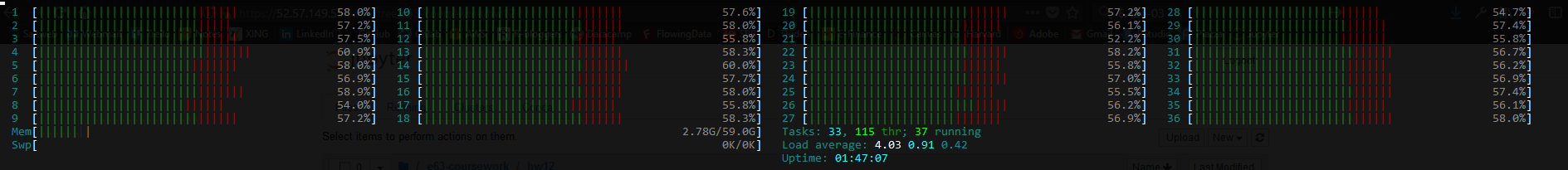# Problem 1 (45%)¶

Please find attached 2 files from Google’s tutorials sets. I used file mnist2.py for preparation of my notes. If you read the file carefully you will see that you can run it in at least two modes. The way it is setup now it selects one learning rate and one particular neural network architecture and generates TensorBoard graph in a particular directory. One problem with this script is that its accuracy is surprisingly low. Such complex architecture and so many lines of code and we get 70% or lower accuracy. We expected more from Convolutional Neural Networks. File cnn_mnist.py is practically the same, at least it does all the same things, creates similar architecture, sets the same or similar parameters, but does a much better job. Its accuracy is in high 90%-s.

Question: Run two files, compare results and then fix the first file (mnist.py) based on what you saw in file cnn_mnist.py.

mnist2.py

The first step is to run the mnist2.py file.

In :
%run /home/tim/e63-coursework/hw12/mnist2.py

Successfully downloaded train-images-idx3-ubyte.gz 9912422 bytes.
Extracting log3/data/train-images-idx3-ubyte.gz
Extracting log3/data/train-labels-idx1-ubyte.gz
Extracting log3/data/t10k-images-idx3-ubyte.gz
Extracting log3/data/t10k-labels-idx1-ubyte.gz
Starting run for lr_1E-04conv2fc2


Next I'm running tensorgraph to visualize the results.

tensorboard --logdir=log3/lr_1E-04conv2fc2

In :
fig = Image(filename=('/home/tim/e63-coursework/hw12/img/p1_1.png'))
fig

Out: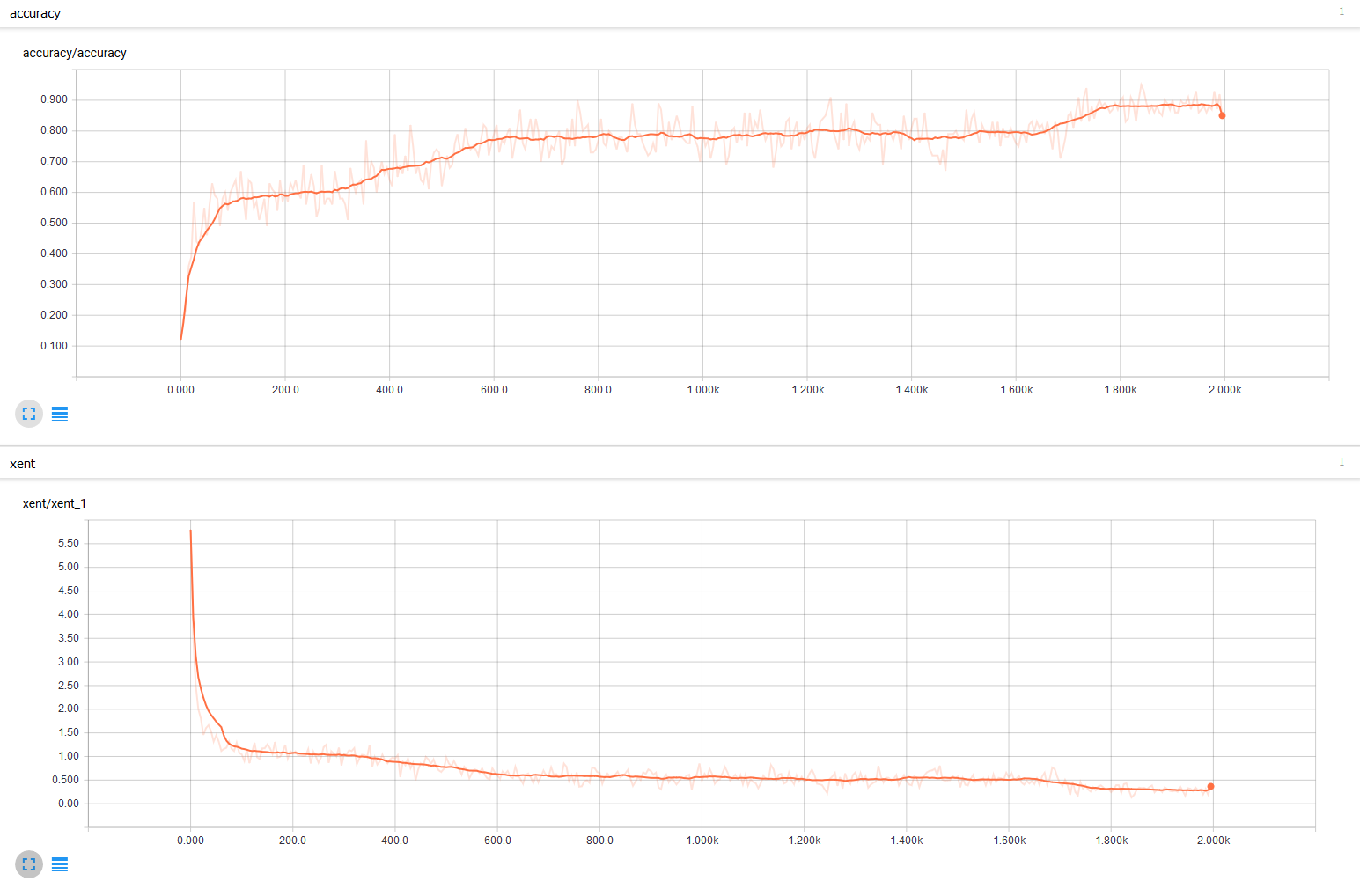The above plot shows, that accuracy rate is at 85%. This accuracy rate is a bit higher than descibed in the problem description. Nevertheless, with such complex architecture and so many lines of code and we would expect a higher rate.

cnn_mnist.py

We can also run the cnn_mnist.py model

In :
%run /home/tim/e63-coursework/hw12/cnn_mnist.py

Successfully downloaded train-images-idx3-ubyte.gz 9912422 bytes.
Extracting temp/train-images-idx3-ubyte.gz
Extracting temp/train-labels-idx1-ubyte.gz
Extracting temp/t10k-images-idx3-ubyte.gz
Extracting temp/t10k-labels-idx1-ubyte.gz
Generation # 5. Train Loss: 2.25. Train Acc (Test Acc): 20.00 (23.60)
Generation # 10. Train Loss: 2.07. Train Acc (Test Acc): 29.00 (31.60)
Generation # 15. Train Loss: 1.94. Train Acc (Test Acc): 44.00 (52.40)
Generation # 20. Train Loss: 1.57. Train Acc (Test Acc): 65.00 (57.20)
Generation # 25. Train Loss: 1.40. Train Acc (Test Acc): 62.00 (66.60)
Generation # 30. Train Loss: 1.08. Train Acc (Test Acc): 72.00 (75.40)
Generation # 35. Train Loss: 0.84. Train Acc (Test Acc): 77.00 (78.00)
Generation # 40. Train Loss: 0.65. Train Acc (Test Acc): 84.00 (83.00)
Generation # 45. Train Loss: 0.57. Train Acc (Test Acc): 82.00 (85.00)
Generation # 50. Train Loss: 0.31. Train Acc (Test Acc): 94.00 (86.60)
Generation # 55. Train Loss: 0.47. Train Acc (Test Acc): 86.00 (86.00)
Generation # 60. Train Loss: 0.32. Train Acc (Test Acc): 90.00 (88.20)
Generation # 65. Train Loss: 0.49. Train Acc (Test Acc): 83.00 (88.60)
Generation # 70. Train Loss: 0.44. Train Acc (Test Acc): 89.00 (90.20)
Generation # 75. Train Loss: 0.30. Train Acc (Test Acc): 91.00 (90.80)
Generation # 80. Train Loss: 0.21. Train Acc (Test Acc): 93.00 (87.80)
Generation # 85. Train Loss: 0.39. Train Acc (Test Acc): 89.00 (90.40)
Generation # 90. Train Loss: 0.37. Train Acc (Test Acc): 89.00 (90.00)
Generation # 95. Train Loss: 0.24. Train Acc (Test Acc): 94.00 (89.80)
Generation # 100. Train Loss: 0.36. Train Acc (Test Acc): 89.00 (91.40)
Generation # 105. Train Loss: 0.34. Train Acc (Test Acc): 92.00 (90.60)
Generation # 110. Train Loss: 0.41. Train Acc (Test Acc): 88.00 (90.20)
Generation # 115. Train Loss: 0.21. Train Acc (Test Acc): 95.00 (91.20)
Generation # 120. Train Loss: 0.37. Train Acc (Test Acc): 89.00 (91.80)
Generation # 125. Train Loss: 0.32. Train Acc (Test Acc): 91.00 (92.00)
Generation # 130. Train Loss: 0.33. Train Acc (Test Acc): 88.00 (93.00)
Generation # 135. Train Loss: 0.38. Train Acc (Test Acc): 93.00 (94.00)
Generation # 140. Train Loss: 0.30. Train Acc (Test Acc): 94.00 (91.20)
Generation # 145. Train Loss: 0.27. Train Acc (Test Acc): 90.00 (91.80)
Generation # 150. Train Loss: 0.18. Train Acc (Test Acc): 94.00 (93.00)
Generation # 155. Train Loss: 0.33. Train Acc (Test Acc): 89.00 (93.40)
Generation # 160. Train Loss: 0.20. Train Acc (Test Acc): 95.00 (90.20)
Generation # 165. Train Loss: 0.24. Train Acc (Test Acc): 92.00 (93.60)
Generation # 170. Train Loss: 0.20. Train Acc (Test Acc): 93.00 (93.80)
Generation # 175. Train Loss: 0.38. Train Acc (Test Acc): 87.00 (94.40)
Generation # 180. Train Loss: 0.14. Train Acc (Test Acc): 95.00 (93.00)
Generation # 185. Train Loss: 0.23. Train Acc (Test Acc): 95.00 (92.40)
Generation # 190. Train Loss: 0.17. Train Acc (Test Acc): 96.00 (94.00)
Generation # 195. Train Loss: 0.30. Train Acc (Test Acc): 92.00 (94.80)
Generation # 200. Train Loss: 0.23. Train Acc (Test Acc): 95.00 (93.80)
Generation # 205. Train Loss: 0.22. Train Acc (Test Acc): 93.00 (93.60)
Generation # 210. Train Loss: 0.32. Train Acc (Test Acc): 94.00 (94.00)
Generation # 215. Train Loss: 0.20. Train Acc (Test Acc): 95.00 (95.80)
Generation # 220. Train Loss: 0.21. Train Acc (Test Acc): 91.00 (95.80)
Generation # 225. Train Loss: 0.22. Train Acc (Test Acc): 93.00 (92.20)
Generation # 230. Train Loss: 0.18. Train Acc (Test Acc): 92.00 (94.80)
Generation # 235. Train Loss: 0.23. Train Acc (Test Acc): 95.00 (94.20)
Generation # 240. Train Loss: 0.20. Train Acc (Test Acc): 95.00 (95.60)
Generation # 245. Train Loss: 0.18. Train Acc (Test Acc): 95.00 (93.20)
Generation # 250. Train Loss: 0.24. Train Acc (Test Acc): 95.00 (94.80)
Generation # 255. Train Loss: 0.30. Train Acc (Test Acc): 92.00 (95.20)
Generation # 260. Train Loss: 0.11. Train Acc (Test Acc): 97.00 (96.00)
Generation # 265. Train Loss: 0.21. Train Acc (Test Acc): 92.00 (92.60)
Generation # 270. Train Loss: 0.11. Train Acc (Test Acc): 97.00 (93.20)
Generation # 275. Train Loss: 0.20. Train Acc (Test Acc): 93.00 (94.60)
Generation # 280. Train Loss: 0.24. Train Acc (Test Acc): 92.00 (95.60)
Generation # 285. Train Loss: 0.23. Train Acc (Test Acc): 93.00 (94.80)
Generation # 290. Train Loss: 0.13. Train Acc (Test Acc): 97.00 (95.80)
Generation # 295. Train Loss: 0.10. Train Acc (Test Acc): 96.00 (93.40)
Generation # 300. Train Loss: 0.18. Train Acc (Test Acc): 96.00 (92.00)
Generation # 305. Train Loss: 0.18. Train Acc (Test Acc): 94.00 (93.40)
Generation # 310. Train Loss: 0.09. Train Acc (Test Acc): 97.00 (94.60)
Generation # 315. Train Loss: 0.14. Train Acc (Test Acc): 95.00 (95.40)
Generation # 320. Train Loss: 0.11. Train Acc (Test Acc): 97.00 (95.80)
Generation # 325. Train Loss: 0.11. Train Acc (Test Acc): 98.00 (95.20)
Generation # 330. Train Loss: 0.16. Train Acc (Test Acc): 95.00 (95.80)
Generation # 335. Train Loss: 0.12. Train Acc (Test Acc): 95.00 (96.40)
Generation # 340. Train Loss: 0.15. Train Acc (Test Acc): 96.00 (96.00)
Generation # 345. Train Loss: 0.21. Train Acc (Test Acc): 94.00 (96.60)
Generation # 350. Train Loss: 0.08. Train Acc (Test Acc): 97.00 (95.80)
Generation # 355. Train Loss: 0.18. Train Acc (Test Acc): 94.00 (97.00)
Generation # 360. Train Loss: 0.19. Train Acc (Test Acc): 95.00 (94.40)
Generation # 365. Train Loss: 0.11. Train Acc (Test Acc): 97.00 (96.00)
Generation # 370. Train Loss: 0.18. Train Acc (Test Acc): 95.00 (96.40)
Generation # 375. Train Loss: 0.17. Train Acc (Test Acc): 94.00 (96.80)
Generation # 380. Train Loss: 0.16. Train Acc (Test Acc): 94.00 (95.20)
Generation # 385. Train Loss: 0.09. Train Acc (Test Acc): 99.00 (96.60)
Generation # 390. Train Loss: 0.20. Train Acc (Test Acc): 93.00 (94.20)
Generation # 395. Train Loss: 0.12. Train Acc (Test Acc): 94.00 (95.80)
Generation # 400. Train Loss: 0.08. Train Acc (Test Acc): 98.00 (96.40)
Generation # 405. Train Loss: 0.10. Train Acc (Test Acc): 98.00 (95.80)
Generation # 410. Train Loss: 0.04. Train Acc (Test Acc): 99.00 (94.80)
Generation # 415. Train Loss: 0.16. Train Acc (Test Acc): 94.00 (96.20)
Generation # 420. Train Loss: 0.17. Train Acc (Test Acc): 94.00 (95.40)
Generation # 425. Train Loss: 0.13. Train Acc (Test Acc): 98.00 (96.60)
Generation # 430. Train Loss: 0.10. Train Acc (Test Acc): 98.00 (94.80)
Generation # 435. Train Loss: 0.12. Train Acc (Test Acc): 98.00 (95.40)
Generation # 440. Train Loss: 0.20. Train Acc (Test Acc): 93.00 (94.00)
Generation # 445. Train Loss: 0.26. Train Acc (Test Acc): 91.00 (94.00)
Generation # 450. Train Loss: 0.10. Train Acc (Test Acc): 97.00 (93.80)
Generation # 455. Train Loss: 0.16. Train Acc (Test Acc): 93.00 (96.20)
Generation # 460. Train Loss: 0.08. Train Acc (Test Acc): 99.00 (96.40)
Generation # 465. Train Loss: 0.14. Train Acc (Test Acc): 97.00 (95.60)
Generation # 470. Train Loss: 0.20. Train Acc (Test Acc): 94.00 (96.00)
Generation # 475. Train Loss: 0.07. Train Acc (Test Acc): 97.00 (97.20)
Generation # 480. Train Loss: 0.12. Train Acc (Test Acc): 96.00 (95.60)
Generation # 485. Train Loss: 0.06. Train Acc (Test Acc): 98.00 (96.60)
Generation # 490. Train Loss: 0.12. Train Acc (Test Acc): 96.00 (97.00)
Generation # 495. Train Loss: 0.12. Train Acc (Test Acc): 96.00 (96.40)
Generation # 500. Train Loss: 0.11. Train Acc (Test Acc): 97.00 (96.40)


The above output shows, that the accuracy rate on test data lies at 95%. As described in the problem statment, this value is better than the result from the mnist2.py model.

Comparison

When comparing the two codes we can see that there are a lot of differences between them:

• Different Learning rates
• Initialization values for the convolution layers
• Dimension of the filters
• The Optimization algorithm (Adam vs. Momentum)
• Number of features in the first layer of convolution
• Number of features in the second layer of convolution
• Number of features in the input to first fully connected layer
• Number of features in the output of first fully connected layer

However, a likely culprit is that the dropout in order to avoid overfitting is missing in the mnist2.py script. That means the mnist2.py could be overfitting the data and the accuracy can't get into the same heights as the cnn_mnist.py script. I did fix this with the following lines of code:

First on line 61 I constructed a placeholder for the dropout.

keep_prob = tf.placeholder(tf.float32)


Next on line 79 I applied the dropout.

fc1_drop = tf.nn.dropout(fc1, keep_prob)
logits = fc_layer(fc1_drop, 1024, 10, "fc2")


Then on line 124 and 131 I'm feeding the probabilities:

[train_accuracy, s] = sess.run([accuracy, summ],
feed_dict={x: batch,y: batch,
keep_prob: 1.0})
sess.run(train_step, feed_dict={x: batch,
y: batch,
keep_prob: 0.5})


Note: I also fixed the log file location inside the script. The fixed working code can be found in the p1_mnist2.py file.

Question: Capture the Accuracy and Cross Entropy (summary) graphs from the corrected version of mnist2.py and provide working and fixed version of that file.

In :
%run /home/tim/e63-coursework/hw12/p1_mnist2.py

Successfully downloaded train-images-idx3-ubyte.gz 9912422 bytes.
Extracting log3_fixeddata/train-images-idx3-ubyte.gz
Extracting log3_fixeddata/train-labels-idx1-ubyte.gz
Extracting log3_fixeddata/t10k-images-idx3-ubyte.gz
Extracting log3_fixeddata/t10k-labels-idx1-ubyte.gz
Starting run for lr_1E-04conv2fc2


Next I'm running tensorgraph to visualize the results.

tensorboard --logdir=log3_fixedlr_1E-04conv2fc2

In :
fig = Image(filename=('/home/tim/e63-coursework/hw12/img/p1_3.png'))
fig

Out: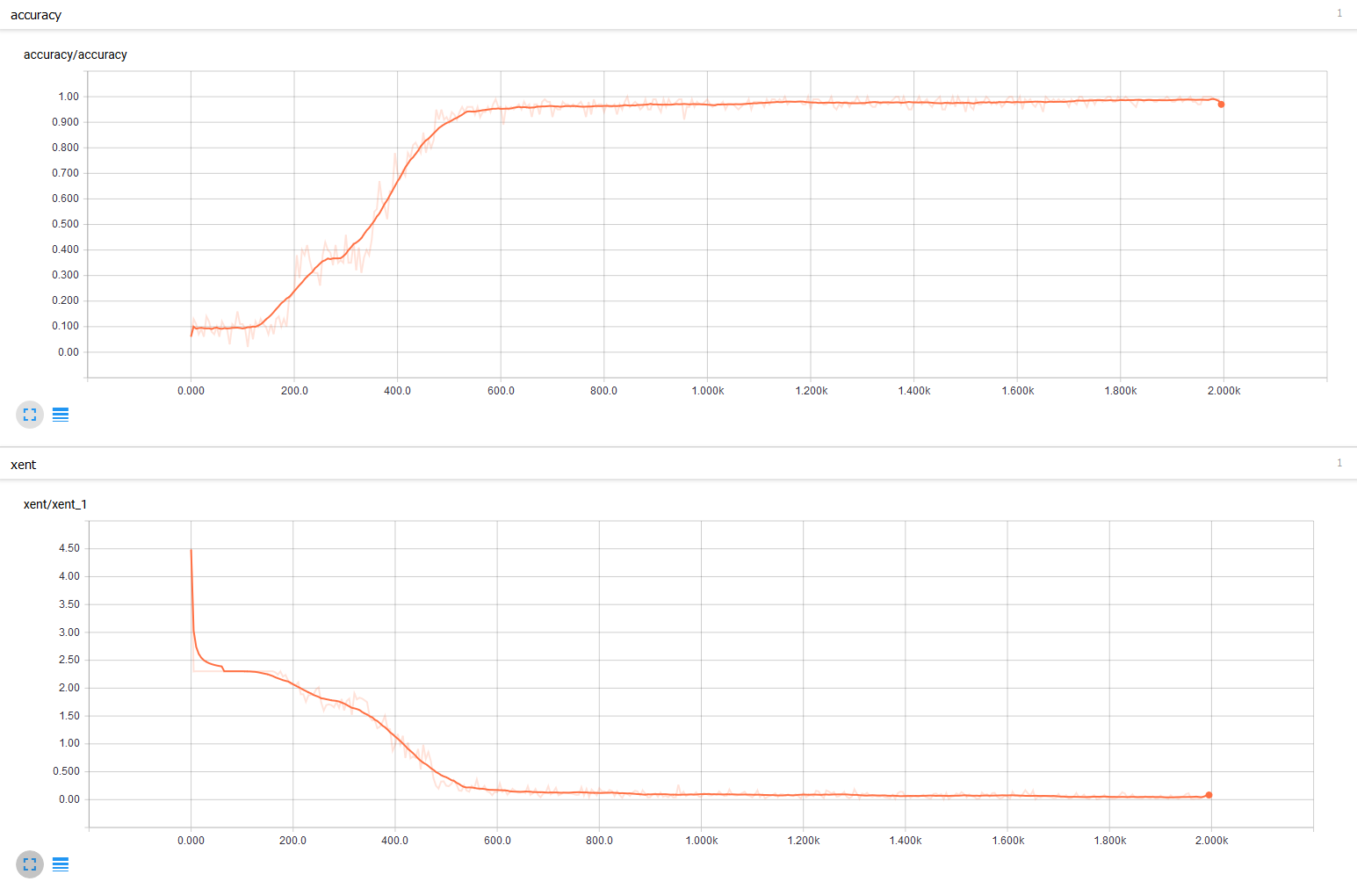With the fixed script the accuracy rate heavily increases and the cross entropy rate decreases. With an accuracy rate of 97% I'm really happy and leave the model be. The model graph looks as follows:

In :
fig = Image(filename=('/home/tim/e63-coursework/hw12/img/p1_4.png'))
fig

Out: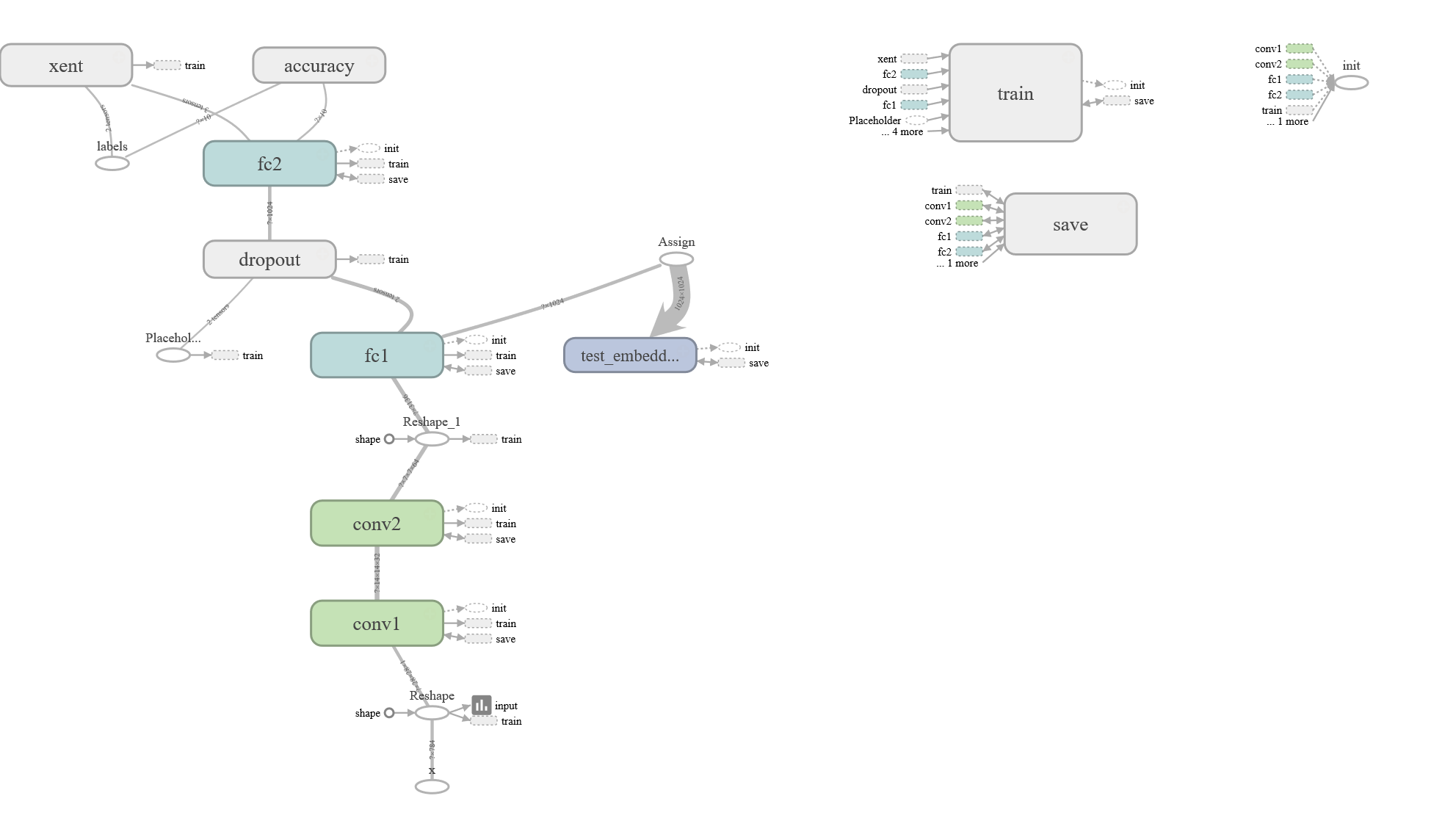# Problem 2 (20%)¶

Question: Run a corrected version of mnist2.py for 4 different architectures (2 conv, 1 conv, 2 fully connected, 1 fully connected layer) and 3 values of the learning rate. As one learning rate choose the one you selected in Problem 1 and then add one smaller and one larger learning rate around that one.

First I'm starting adapting the mnist2.py script. All I'm doing is to change the main() function at the end of the script. The number of convolutional layers, the number of FC layers and the following learning rates are beeing set: 1E-03 (1'000), 1E-04 (10'000), 1E-05 (100'000). The results of this are saved in the p2_mnist2.py script.

With the following command we're running the p2_mnist2.py script:

In :
%run /home/tim/e63-coursework/hw12/p2_mnist2.py

Successfully downloaded train-images-idx3-ubyte.gz 9912422 bytes.
Extracting log3/data/train-images-idx3-ubyte.gz
Extracting log3/data/train-labels-idx1-ubyte.gz
Extracting log3/data/t10k-images-idx3-ubyte.gz
Extracting log3/data/t10k-labels-idx1-ubyte.gz
Starting run for lr_1E-03conv2fc2
Starting run for lr_1E-03conv1fc2
Starting run for lr_1E-03conv2fc1
Starting run for lr_1E-03conv1fc1
Starting run for lr_1E-04conv2fc2
Starting run for lr_1E-04conv1fc2
Starting run for lr_1E-04conv2fc1
Starting run for lr_1E-04conv1fc1
Starting run for lr_1E-05conv2fc2
Starting run for lr_1E-05conv1fc2
Starting run for lr_1E-05conv2fc1
Starting run for lr_1E-05conv1fc1


Question: Please capture an image of “colorful” T-SNE Embedding.

In order to have a look at the T-SNE Embedding, I'm running the following command:

tensorboard --logdir=log3/lr_1E-03conv2fc2

In :
from IPython.display import Image
fig = Image(filename=('/home/tim/e63-coursework/hw12/img/p2_img1.png'))
fig

Out: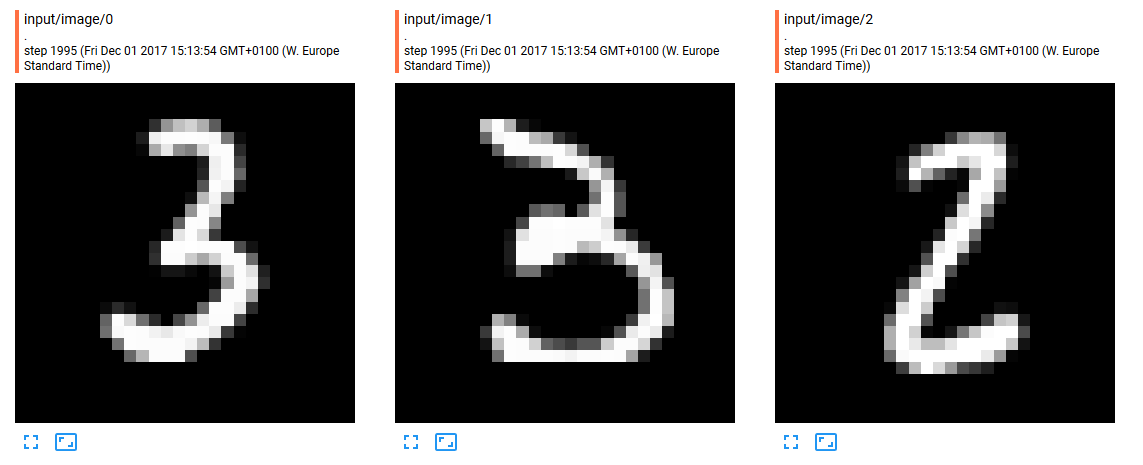In :
fig = Image(filename=('/home/tim/e63-coursework/hw12/img/p2_img2.png'))
fig

Out: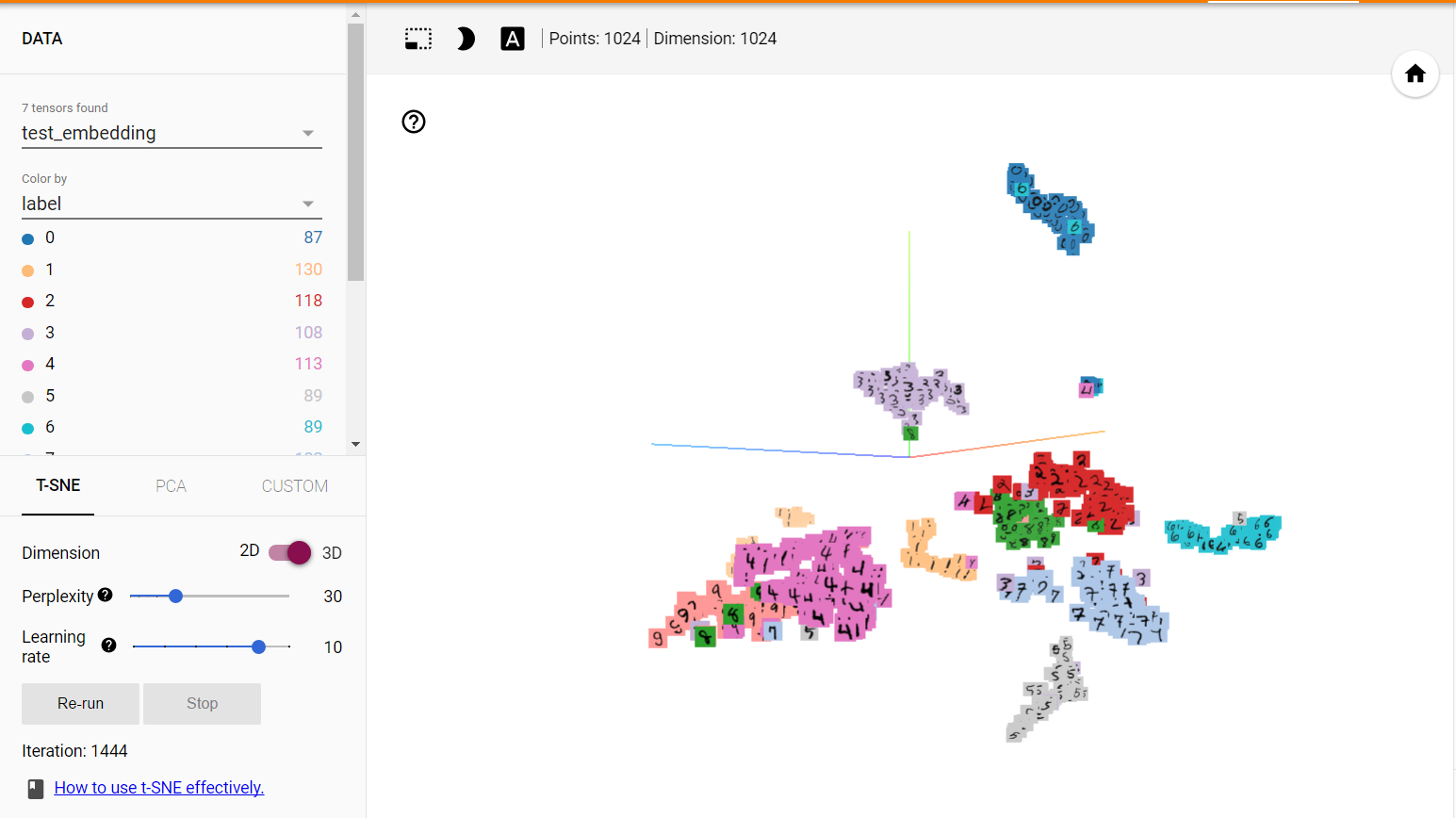Question: Capture Accuracy (summary) graphs and one of the Histograms to demonstrate to us that your code is working.

As above, I'm demonstrate that the code is working with conv2fc2. We're starting with the smallest learning rate and move on to the bigger ones.

Learning rate: 1E-03 (1'000)

In :
fig = Image(filename=('/home/tim/e63-coursework/hw12/img/p2_conv2fc2_1e03_1.png'))
fig

Out: